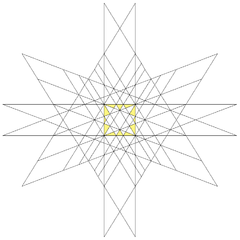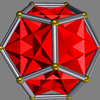# Compound of five cubes

﻿
Compound of five cubes
Compound of five cubesType Regular compound
Stellation core rhombic triacontahedron
Convex hull Dodecahedron
Index UC9
Polyhedra 5 cubes
Faces 30 squares
Edges 60
Vertices 20
Dual Compound of five octahedra
Symmetry group icosahedral (Ih)
Subgroup restricting to one constituent pyritohedral (Th)

This polyhedral compound is a symmetric arrangement of five cubes. This compound was first described by Edmund Hess in 1876.

It is one of five regular compounds, and dual to the compound of five octahedra.

It is one of the stellations of the rhombic triacontahedron. It has icosahedral symmetry (Ih).

Its convex hull is a regular dodecahedron. It additionally shares its edge arrangement with the small ditrigonal icosidodecahedron, the great ditrigonal icosidodecahedron, and the ditrigonal dodecadodecahedron.

The compound of ten tetrahedra can be formed by taking each of these five cubes and replacing them with the two tetrahedra of the Stella octangula (which share the same vertex arrangement of a cube).

## As a stellation

This compound can be formed as a stellation of the rhombic triacontahedron. The 30 rhombic faces exist in the planes of the 5 cubes.

The stellation facets for construction are:## As a facettingFive cubes in a dodecahedron

It is also a faceting of a dodecahedron, shown at left, and is also a faceting of the icosidodecahedron.